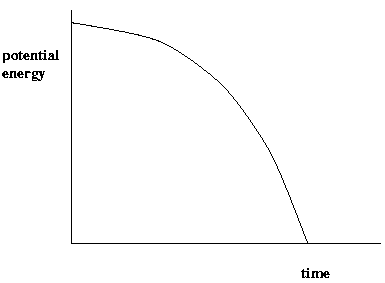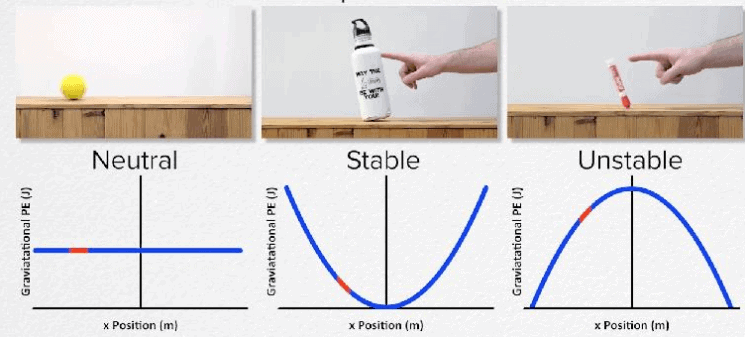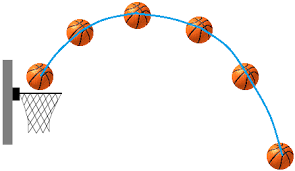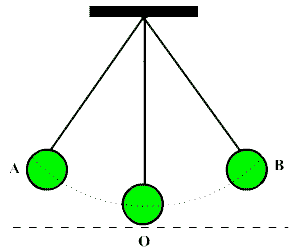UPSC  >  Work, Energy, Power, Simple Pendulum & Restoring Force

# Work, Energy, Power, Simple Pendulum & Restoring Force - Notes | Study Science & Technology for UPSC CSE - UPSC

 1 Crore+ students have signed up on EduRev. Have you?

Work: Work is said to be done when the point of application of a force is moved through a distance. The unit of work in the S.I. system is the Joule and is the work done when the point of application of a force of one Newton is moved through a distance of one metre in the direction of the force.

The erg: The erg is the unit of work in the C.G.S. system and is the work.

Energy: Energy is the capacity for doing work and is expressed in the same units as work. It is of two kinds namely, kinetic energy and potential energy. Kinetic energy is the energy possessed by a body by virtue of its motion.

Potential Energy:Potential energy is energy possessed by a body by virtue of its position. When a body is at an elevated position with respect to the ground level, it possesses potential energy.

 Energy Among non-conventional energy sources, the potential of wind energy is the highest of all.The first use of solar photo-voltaic cells was made by the US in 1958 for space missions.Australia was the first to develop solar cooker, which was as efficient as electric or gas stove.In India, solar energy was initially used for cooking purpose in Jamshedpur.The target for sale of solar cookers by 1992-93 was 40,000.The solar pond was initially developed in Israel as a cheap device for collecting solar energy on a large scale for supplying thermal energy.Solar ponds possess an inherent energy storage capacity because of high specific heat of water.The world’s largest solar pond is in India at Madhapar, near Bhuj, Kutch (Gujarat) on an area of 60,000 sq. metres and a depth of 12 feet, providing 80,000 litres of hot water at 70°C.An important application of solar ponds is to get potable water.The world’s first solar electric village, Schuchuli, in Arizona, was lighted with solar cells.In 1989, a solar power plant was set up at the solar energy centre near Gurgadu in Haryana.Solar energy is already being used in lighting the entire civil airport area near Udaipur (Rajasthan).The Gujarat Energy Development Association (GEDA) hosted the first and second national workshops on solar pond in Baroda city in 1983 and 1985.Up flow Anaerobic Sludge Blanket (UASB) is the best and most favoured technology globally for generating energy from biodegradable wastes.During 8th plan in India 500 MW of Wind energy would be generated and about Rs. 857 crores has been allocated by the Planning Commission for this purpose.The centre is also considering the World Bank master plan to create Wind Power Plants of 640 MW in Andhra Pradesh and 700 MW in Tamil Nadu.The “roller compacted concrete” (RCC) technology of dam construction was evolved in the eighties to combine economical and rapid placing techniques of embankment materials.

Power: Power is the ratio of doing work and is measured in watts. If an engine is doing work at the rate of 1 joule (1J) per sec, its power is one watt. Centre of gravity can be defined as the point at which the whole weight of the body may be supposed to act.

The centre of gravity need not in all cases be located inside the body; it can be outside too in some cases. Thus in the case of a ring, it is located at the centre of the ring which is outside the material of the ring. The centre of gravity of a circular lamina is at its centre, of a triangular lamina it is at the point of a trisection of the medians and of square, rectangular lamina, at the point of intersection of the diagonals.

EquilibriumFig. Different types of equilibrium

Equilibrium means the state of rest. It is of 3 types viz.,

(i) stable equilibrium

(ii) unstable equilibrium

(iii) neutral equilibrium.

Stable equilibrium: A body is in stable equilibrium when its centre of gravity is low and any slight tilting of the body raises the centre of gravity.
A funnel resting on its mouth on the table is an instance in point. In such bodies the vertical line from the centre of gravity will fall within the base of support.

Unstable equilibrium: A body in unstable equilibrium will have a high centre of gravity. If tilted it a little the C.G. will be lowered. And the vertical line drawn from it (the C.G.) will fall outside the base of support and so the body will easily topple over.
A funnel resting vertically at the bottom end of its stem on the table with its mouth facing upward is in unstable equilibrium.

Neutral equilibrium: In this case the centre of gravity remains at the same height neither raised nor lowered when body is titled.

A funnel resting on its sides illustrates this type. People bend forward while carrying loads on their backs. Table lamps are made with heavy bases. While crossing rivers in boats, people sit down and do not stand. Racing cars are wide at the wheels and built low. These are to create conditions for more stability.
Gravitation

Force of gravitation. The force of attraction which exists between any two objects in the universe is called as the force of gravitation.

Gravity. It is a force of attraction exerted by the earth (or planet) on all the bodies lying on or near its surface. Gravity is a special case of Gravitation.

Universal character of gravitational force. The force of gravitation is universal in character because the force exists between any two objects situated anywhere in the universe.Fig. Gravitational force around earth due to sun

Newton’s law of gravitation. The law states that any two bodies in the universe attract each other with a force whose magnitude is directly proportional to the product of their masses and inversely proportional to the square of the distances between them. The direction of the force is along the line joining the two masses.
Universal gravitational constant ‘G’ can be defined as that force of gravitation which is exerted mutually between two bodies each of unit mass and separated from one another by a unit distance.

Unit of G- In c.g.s. system the value of G is 6.673 * 10-2 dyn cm2g and in SI units the value of G is  6.673 x10-11 Nm2 kg-2.

According to Newton’s third law of motion, whenever a force is exerted by an object on another object, second object also exerts an equal force on the first object.
The above holds good for gravitation also. If a stone falls on the earth due to gravitational force, earth should also move towards the stone, because stone also attracts the earth with an equal force. But no such rise of earth towards the stone is observed, because if the mass is large its acceleration will be small. Since the mass of earth is very large as compared to stone, its acceleration is too small to be observed and hence we are not able to see its rise towards the stone.

Acceleration due to gravity ‘g’. All bodies irrespective of their masses fall towards the earth with the same acceleration (in the absence of air), which is known as acceleration due to gravity and is denoted by ‘g’.

Value of ‘g’ on earth = 9.8 ms-2

and its value on moon =1g/6

Inertial mass. The mass of a body which measures its inertia and which is given by the ratio of external force to the acceleration produced in it  is called inertial mass.

Gravitational mass. The mass of a body which determines the gravitational pull due to earth (or any other body) acting upon it is called gravitational mass. The two objects will be said to possess the same gravitational mass, if the forces of earth’s gravity on them are equal; provided both the objects are placed at the same distance from the centre of the earth.

Weight. Weight of an object is defined as force with which it is attracted towards the centre of earth.

Variation of ‘g’

(i) Due to shape of the earth: The value of ‘g’ at the pole is greater than that at the equator.

(ii) Due to rotation of the earth: Value of ‘g’ is least at the equator and greatest at the pole.

(iii) Due to altitudes: Value of ‘g’ decreases as we go away from the surface of the earth. (iv) Inside the earth: Value of ‘g’ decreases as we go towards the centre inside the earth.

 Difference between g and G Acceleration due to gravity “g” Universal gravitational constant “G” (i) It is the acceleration acquired by a body due to earth's gravitational pull on it.(ii) "g” is not a universal constant. It is different at different placeson the surface of the earth. Its value varies from one celestial body to another.(iii) It is a vector quantity. (i) It is numerically equal to the force of attraction between two masses of 1 kg each separated by a distance of 1m.(ii) “G” is a universal constant, i.e., its value is same viz., 6.7 x 10-11Nm2 kg-2 every where in the universe.(iii)  It is a scalar quantity.

Mass and Density of the Earth

Mass = 6 *1024 kg (Approx.)

Density E = 5.5 ´ 10kg/m3

Radius = 6.37 * 106 m.

While standing in a lift, a man feels

(i) heavy, when lift goes upward with a constant acceleration,

(ii) light, when lift is moving down with a constant acceleration,

(iii) weightless, when lift is falling freely.

The weight of a body would be more if the earth stopped rotating.

Conversely, if the speed of rotation were higher, the weight would be less. It is not hard to imagine that at critical speed of rotation of the earth a body would become weight less.

Escape Velocity is the least velocity required to throw a body from the surface of the earth so that it may not return. Escape Velocity =  (√(2gr)) where R is the Radius of the earth. Escape Velocity is 11.2 km/sec. or, 7 miles/sec.

A Geostationary or, Synchronous Satellites is one which appears stationary with respect to the earth. The period of rotation of the earth about its axis is 24 hours.
Thus if a satellite orbiting the earth over the equator has a 24-hour period of revolution, it appear stationary. The 24-hour time period is possible when a satellite is at a height of nearly 35,000 km above the earth. These satellites (like that of India’s INSAT-1D) are used for communication and weather forecasting.

Astronaut in space is weightless. The space vehicle, astronaut and scale all fall towards the earth with same acceleration. So the astronaut does not exert any force on the scale or the sides of vehicle and, therefore, is weightless.

Projectile:fig. Projectile motionAn object which is thrown at certain angle with the horizontal is called a projectile. The path followed by a projectile is called trajectory. The maximum horizontal distance is called range.

Some important points:

(i) Trajectory of a projectile is curved because of gravitational attraction.

(ii) All projectiles thrown horizontally and simultaneously from a point with different initial speeds reach the ground at the same time.

(iii) All projectiles thrown horizontally and simultaneously from a point with different initial speeds cover different horizontal distances, distance being more if the speed is more.

A motion in which an object moves back and forth repeatedly about a fixed position is called an oscillatory motion. A motion which repeats itself after equal interval of time, is called a periodic motion.

Simple pendulum. A small heavy body suspended by a light inextensible string is called a simple pendulum.

Length of pendulum. It is the distance between point of suspension and the point of oscillation. It is denoted by L. Length of pendulum = length of string + radius of bob.
The motion of bob from its extreme position from A to B and back to A is called an oscillation.

Periodic time or time periodFig. Oscillation of pendulum It is defined as the time taken to complete one oscillation i.e., it is time taken to travel from one extreme to other and back. It is called time period or simply period. It is denoted by T. The original rest position O of the bob is called mean position or fixed position of the pendulum. The maximum displacement of the pendulum on either side of its mean position is called as the amplitude of oscillations. OA and OB are the amplitudes.The number of oscillations completed by the pendulum in one second is called its frequency.

Damping oscillations. A gradual decrease in the amplitude of oscillation is called damping oscillations. The damping of amplitude for pendulum is due to the air friction and the friction at the support.

Restoring force. Such force which is always directed towards the mean position during an oscillatory motion is called the restoring force. The oscillation of a mass on a spring is an example of restoring force.

Origin of restoring force on pendulum: It is the combination of the two forces acting on the bob viz., the gravitational pull of the earth and the force of tension in the string which gives rise to the restoring force directed towards the mean position.

The document Work, Energy, Power, Simple Pendulum & Restoring Force - Notes | Study Science & Technology for UPSC CSE - UPSC is a part of the UPSC Course Science & Technology for UPSC CSE.
All you need of UPSC at this link: UPSC

## Science & Technology for UPSC CSE

86 videos|351 docs|235 tests
 Use Code STAYHOME200 and get INR 200 additional OFF

## Science & Technology for UPSC CSE

86 videos|351 docs|235 tests

### How to Prepare for UPSC

Read our guide to prepare for UPSC which is created by Toppers & the best Teachers

Track your progress, build streaks, highlight & save important lessons and more!

,

,

,

,

,

,

,

,

,

,

,

,

,

,

,

,

,

,

,

,

,

,

,

,

,

,

,

,

,

,

;